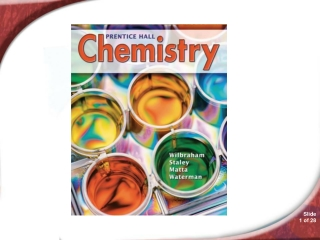DownloadDownload PresentationChemistry 18.4

# Chemistry 18.4

Download Presentation## Chemistry 18.4

- - - - - - - - - - - - - - - - - - - - - - - - - - - E N D - - - - - - - - - - - - - - - - - - - - - - - - - - -
##### Presentation Transcript

1. Chemistry 18.4

2. 18.4 Entropy and Free Energy • Inside a pile of oily rags or a stack of hay that has not been thoroughly dried, decomposition causes heat to build up. When heat cannot escape, the temperature can become high enough to cause a fire. You will learn about the conditions that will produce a spontaneous chemical reaction.

3. 18.4 Free Energy and SpontaneousReactions • Free Energy and Spontaneous Reactions • What are two characteristics of spontaneous reactions?

4. 18.4 Free Energy and SpontaneousReactions • A spontaneous reaction occurs naturally and favors the formation of products at the specified conditions.

5. 18.4 Free Energy and SpontaneousReactions • A nonspontaneous reaction is a reaction that does not favor the formation of products at the specified conditions. Photosynthesis is a nonspontaneous reaction that requires an input of energy.

6. 18.4 Free Energy and SpontaneousReactions • Spontaneous reactions produce substantial amounts of products at equilibrium and release free energy. • Free energy is energy that is available to do work.

7. 18.4 Entropy • Entropy • What part does entropy play in chemical reactions?

8. 18.4 Entropy • Entropy is a measure of the disorder of a system. • Physical and chemical systems attain the lowest possible energy. • The law of disorder states that the natural tendency is for systems to move in the direction of maximum disorder or randomness.

9. 18.4 Entropy • An increase in entropy favors the spontaneous chemical reaction; a decrease favors the nonspontaneous reaction.

10. 18.4 Entropy • For a given substance, the entropy of the gas is greater than the entropy of the liquid or the solid. Similarly, the entropy of the liquid is greater than that of the solid.

11. 18.4 Entropy • Entropy increases when a substance is divided into parts.

12. 18.4 Entropy • Entropy tends to increase in chemical reactions in which the total number of product molecules is greater than the total number of reactant molecules.

13. 18.4 Entropy • Entropy tends to increase when temperature increases. As the temperature increases, the molecules move faster and faster, which increases the disorder.

14. 18.4 Enthalpy, Entropy, and Free Energy • Enthalpy, Entropy, and Free Energy • What two factors determine the spontaneity of a reaction?

15. 18.4 Enthalpy, Entropy, and Free Energy • The size and direction of enthalpy changes and entropy changes together determine whether a reaction is spontaneous; that is, whether it favors products and releases free energy.

16. 18.4 Enthalpy, Entropy, and Free Energy

17. Enthalpy, Entropy, and Free Energy • Simulation 25 • Simulate how enthalpy and entropy changes combine to determine the spontaneity of a reaction.

18. 18.4 Gibbs Free-Energy • Gibbs Free-Energy • Is the Gibbs free-energy change positive or negative in a spontaneous process?

19. 18.4 Gibbs Free-Energy • The Gibbs free-energy change is the maximum amount of energy that can be coupled to another process to do useful work. • The numerical value of ΔG is negative in spontaneous processes because the system loses free energy.

20. 18.4 Gibbs Free-Energy

21. 18.4 Gibbs Free-Energy

22. 18.4 Section Quiz. • 18.4

23. 18.4 Section Quiz. • 1. Free energy from a reaction is the amount of energy that is • absorbed by an entropy decrease. • equal to the enthalpy change. • wasted as heat. • available to do work.

24. 18.4 Section Quiz. • 2. Free energy is always available from reactions that are • endothermic. • nonspontaneous. • at equilibrium. • spontaneous.

25. 18.4 Section Quiz. • 3. Choose the correct words for the spaces: Spontaneous reactions produce ________ and substantial amounts of _________ at equilibrium. • free energy, products • no free energy, reactants • free energy, reactants • no free energy, products

26. 18.4 Section Quiz. • 4. Which of the following involves a decrease in entropy? • Natural gas burns. • A liquid freezes. • Dry ice sublimes. • Water evaporates.

27. 18.4 Section Quiz. • 5. A reaction is spontaneous if • enthalpy decreases and entropy increases. • enthalpy increases and entropy increases. • enthalpy decreases and entropy decreases. • enthalpy increases and entropy decreases.

28. 18.4 Section Quiz. • 6. Choose the correct words for the spaces: Gibbs free-energy change is the _________ amount of energy that can be ___________ another process to do useful work. • maximum, coupled to • maximum, duplicated by • spontaneous, coupled to • minimum, duplicated by

29. END OF SHOW